Rational numbers on number line

Chapter 1 Class 8 Rational Numbers
Concept wise

#### Represent 2 on number line.

Step 1 :  Make a line and mark number from −5 to 5.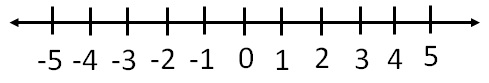Step 2 :    Mark 2.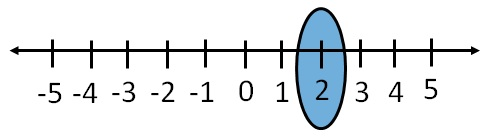#### Represent 12 on number line.

Step 1 :    Make a line and mark number from 0 to 13.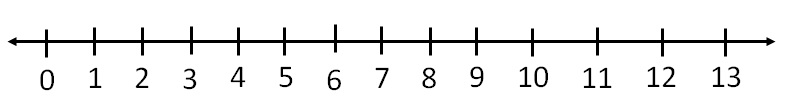Step 2 :  Mark 12.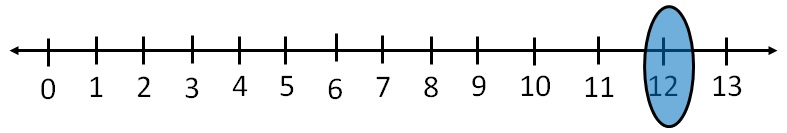#### Represent 1/3  on number line .

Let us draw number line from 0 to 2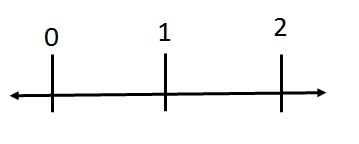Since denominator is 3

∴ We divide 0 & 2 into 3 equal parts.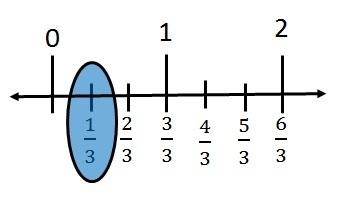#### Represent 7 / 8 on number line .

Let us draw number line from 0 to 2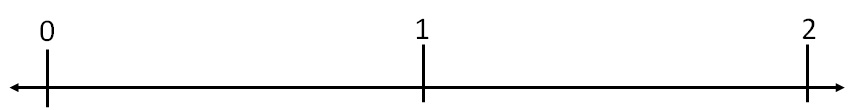Since denominator is 8

∴ We divide 0 & 2 into 8 equal parts.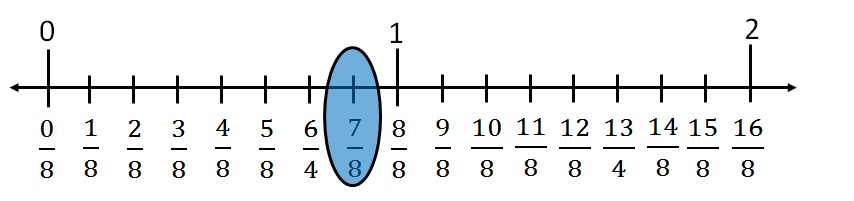#### Represent 2 / 5 , (-4)/5 on number line .

Since (-4)/5 < 0

We draw number line from −1 to 1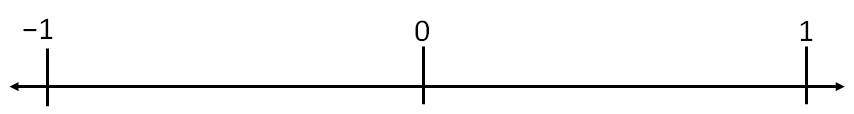Since denominator is 5

∴ We divide number line between 0 & 1 into 5 equal parts.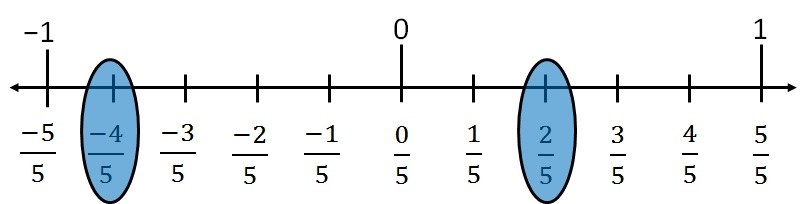Learn in your speed, with individual attention - Teachoo Maths 1-on-1 Class